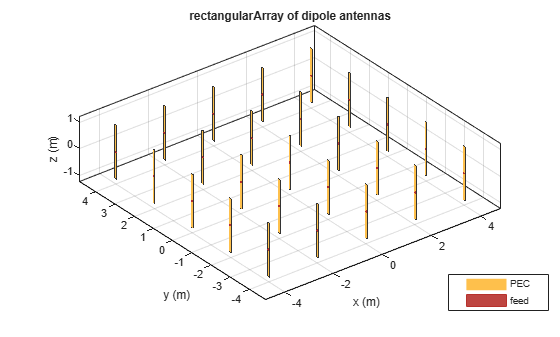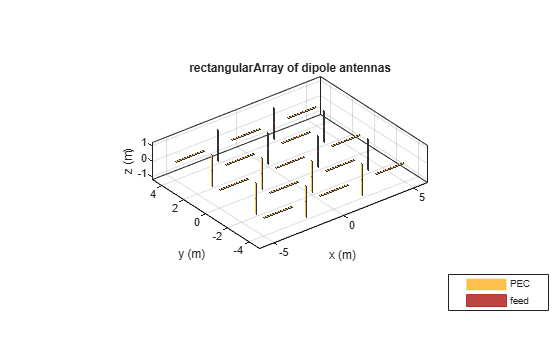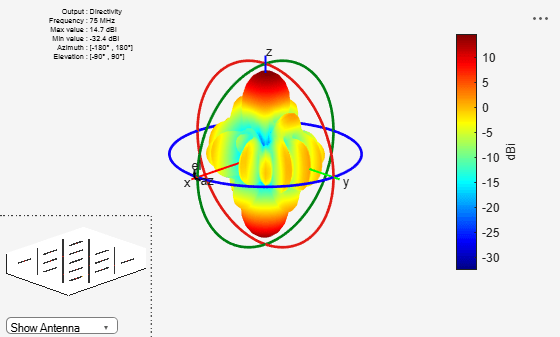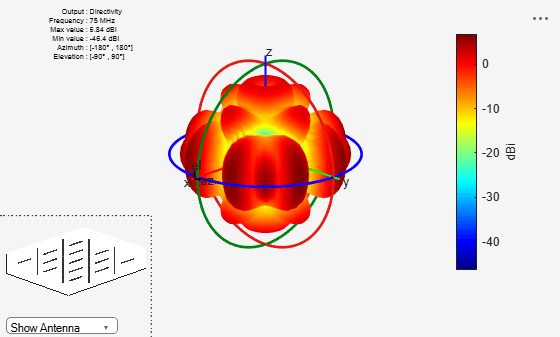# Manipulate Array Elements

This example shows you how to control an individual element in a linear or rectangular array. You can use this technique to change the size and tilt of the antenna, or to model dead elements etc. with individual elements in an array.

### Define Dipole Array

Create a dipole antenna using the `dipole` object. To create a 5-by-5 dipole array, replicate the dipole antenna using a 5-by-5 matrix. Create a rectangular array using the dipole array as a single element.

```d = dipole; N = 5; df = repmat(d,N)```
```df = 5x5 dipole array with properties: Length: {25x1 cell} Width: {25x1 cell} FeedOffset: {25x1 cell} Conductor: {25x1 cell} Tilt: {25x1 cell} TiltAxis: {25x1 cell} Load: {25x1 cell} ```
```r = rectangularArray(Element=df); show(r)```### Tilt Alternate Elements

Create a vector of alternate elements in the rectangular array.

`S = 1:2:N*N;`

Tilt the alternate elements in the array by 90 degrees about the y-axis.

```for i = 1:25 if any(S==i) r.Element(i).Tilt = 90; r.Element(i).TiltAxis = [0 1 0]; end end show(r)```### Pattern of Rectangular Array

Plot the pattern of the array at 75 MHz.

`pattern(r,75e6)`Antennas with a zero excitation voltage feed are called dead elements. By default, each element in an array is excited by an amplitude of 1 V.

`Vfeed = ones(1,N*N)`
```Vfeed = 1×25 1 1 1 1 1 1 1 1 1 1 1 1 1 1 1 1 1 1 1 1 1 1 1 1 1 ```

To model dead elements, set the voltage to zero for all horizontal elements. To control voltage, use the `AmplitudeTaper` property. This property is the excitation amplitude of the antennas in an array.

`Vfeed(S) = 0`
```Vfeed = 1×25 0 1 0 1 0 1 0 1 0 1 0 1 0 1 0 1 0 1 0 1 0 1 0 1 0 ```
`r.AmplitudeTaper = Vfeed`
```r = rectangularArray with properties: Element: [5x5 dipole] Size: [5 5] RowSpacing: 2 ColumnSpacing: 2 Lattice: 'Rectangular' AmplitudeTaper: [0 1 0 1 0 1 0 1 0 1 0 1 0 1 0 1 0 1 0 1 0 1 0 1 0] PhaseShift: 0 Tilt: 0 TiltAxis: [1 0 0] ```

Plot the radiation pattern of the array.

```figure pattern(r,75e6)```